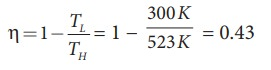Home | | Physics 11th std | Solved Example Problems for Heat Engine

# Solved Example Problems for Heat Engine

Physics : Heat and Thermodynamics - Solved Example Problems for Heat Engine

### EXAMPLE 8.24

During a cyclic process, a heat engine absorbs 500 J of heat from a hot reservoir, does work and ejects an amount of heat 300 J into the surroundings (cold reservoir). Calculate the efficiency of the heat engine?

### Solution

The efficiency of heat engine is given by╬╝ = 1 ŌĆō 0.6 = 0.4

The heat engine has 40% efficiency, implying that this heat engine converts only 40% of the input heat into work.

### EXAMPLE 8.25

a) A steam engine boiler is maintained at 250┬░C and water is converted into steam. This steam is used to do work and heat is ejected to the surrounding air at temperature 300K. Calculate the maximum efficiency it can have?

### Solution

The steam engine is not a Carnot engine, because all the process involved in the steam engine are not perfectly reversible. But we can calculate the maximum possible efficiency of the steam engine by considering it as a Carnot engine.The steam engine can have maximum possible 43% of efficiency, implying this steam engine can convert 43% of input heat into useful work and remaining 57% is ejected as heat. In practice the efficiency is even less than 43%.

### EXAMPLE 8.26

There are two Carnot engines A and B operating in two different temperature regions. For Engine A the temperatures of the two reservoirs are 150┬░C and 100┬░C. For engine B the temperatures of the reservoirs are 350┬░C and 300┬░C. Which engine has lesser efficiency?

### Solution

The efficiency for engine A = 1 ŌłÆ 373/423 = 0.11. Engine A has 11% efficiency

The efficiency for engine B = 1 - 573/623 = 0.08

Engine B has only 8% efficiency.

Even though the differences between the temperature of hot and cold reservoirs in both engines is same, the efficiency is not same. The efficiency depends on the ratio of the two temperature and not on the difference in the temperature. The engine which operates in lower temperature has highest efficiency.

Tags : Thermodynamics , 11th Physics : UNIT 8 : Heat and Thermodynamics
Study Material, Lecturing Notes, Assignment, Reference, Wiki description explanation, brief detail
11th Physics : UNIT 8 : Heat and Thermodynamics : Solved Example Problems for Heat Engine | Thermodynamics